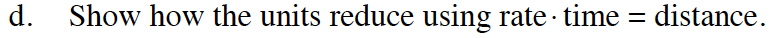### Home > CALC > Chapter 1 > Lesson 1.2.3 > Problem1-55

1-55.
1. A marathon runner runs a 26.2-mile race. Her distance traveled in miles at time t hours is p(t) = 7t. Homework Help ✎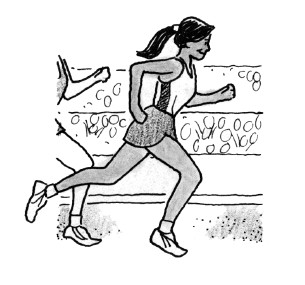1. How long did it take her to finish the race?

2. What was her average velocity? Explain your reasoning.

3. Suppose she runs at a constant pace of 7 miles/hour. How far will she have gone in 2 hours?

4. Show how the units reduce using rate · time = distance.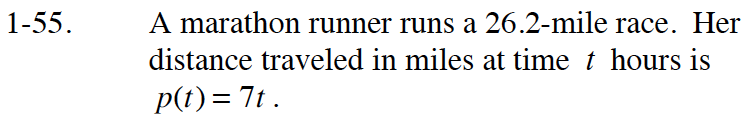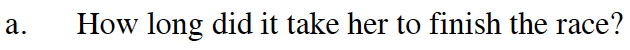26.2 = p(t)
Solve for t.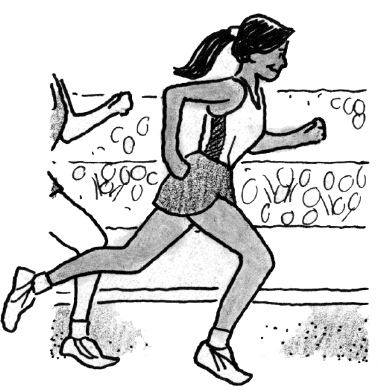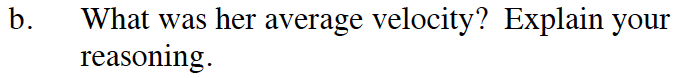$\text{average velocity }= \frac{\Delta \text{ distance}}{\Delta \text{time}}$

Evaluate and explain.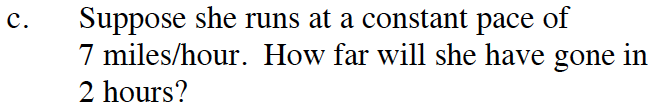$\text{velocity }= \frac{\text{ distance}}{ \text{time}}$

Evaluate.